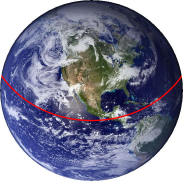Friday, February 11, 2011

The String Around The WorldImagine I had a very long piece of string: long enough to wrap it around the equator of the Earth. And I'll do just that. Yikes — that's about 40,000 kilometers (or 25,000 miles) of string! I will make sure its pulled completely tight and connect both ends to each other: the result is that it lies flat onto the surface.

Now let me extend the string with just one measly meter. Compared to its total length that's not a lot, is it? Once again I pull it tight, but now, with the added meter, it has come off the ground just a tiny bit. Assume that this extra distance (between the string and the Earth's surface) is equally divided and thus the same all around the globe. How much would you guess this distance is? Surely this must be in the order of nanometers or whatnot, right?

Well, no. Turns out, the entire string has now come almost 16 centimeters off the ground.

Counter-intuitive as that might seem, the math behind it is quite clear. The circumference of a circle is calculated as Pi times the diameter of that circle. To put this another way, c = π × r × 2. Grabbing Earth's equatorial radius r = 6378.1 km right of Wikipedia, one can calculate a circumference c = 40,074.7842... km. That's the length of the string whilst flat on the Earth! So now I add that meter (0.001 km) to this number, and calculate back to the radius r. With r = c ÷ (2 × π) one will find 6378.1001591... km. Subtract the starting radius of 6378.1 km and ta-da: the difference is indeed about 15.9 cm.

So changing the circumference of the Earth by just a tiny bit seems to have a massive effect on its radius. Or has it? Maybe look at it differently. The radius of the Earth is thousands of kilometers! In that sense, 15.9 cm is really just a tiny bit. Just as you would expect.

A bit of math trickery might tell you that the above formula can be boiled down to a simple r = 1 ÷ (2 × π). That calculates (in meters) how much the radius of a circle increases when its circumference is extended with 1 meter. But hang on a second. This means that the initial length of the string has no relevance whatsoever!And that's just how it is. Forget about the Earth, and let me place another piece of string around a soccer ball instead. The ball's circumference is 69.85 cm so that's the string's length. Once more I make it 1 meter longer and pull it tight. The distance between the surface of the ball and the string is now... 15.9 cm.

The size of the sphere or circle, it really does not matter.